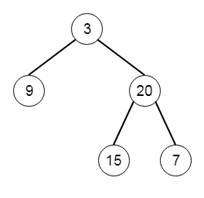# Program to generate tree using preorder and inorder traversal in python

Suppose we have the preorder and inorder traversal of a binary tree. We have to recover the tree from these sequences. So if the preorder and inorder sequences are [3,9,20,15,7] and [9,3,15,20,7], then the tree will be:Let us see the steps:

• Define a method called buildTree(), this will the preorder and inorder traversal sequences.
• if inorder traversal list is not empty, then
• root := create a tree node with value same first element from preorder sequence, and remove first item from preorder traversal
• root_index := index of value of root in inorder list
• left of root := buildTree(preorder, inorder[from index 0 to root_index])
• right of root := buildTree(preorder, inorder[from index root_index+1 to end])
• return root

Let us see the following implementation to get better understanding:

## Example

Live Demo

class TreeNode:
def __init__(self, data, left = None, right = None):
self.data = data
self.left = left
self.right = right

def print_tree(root):
if root is not None:
print_tree(root.left)
print(root.data, end = ',')
print_tree(root.right)

class Solution(object):
def buildTree(self, preorder, inorder):
if inorder:
root = TreeNode(preorder.pop(0))
root_index = inorder.index(root.data)
root.left = self.buildTree(preorder,inorder[:root_index])
root.right = self.buildTree(preorder,inorder[root_index+1:])
return root

ob1 = Solution()
print_tree(ob1.buildTree([3,9,20,15,7], [9,3,15,20,7]))

## Input

[3,9,20,15,7],[9,3,15,20,7]

## Output

9,3,15,20,7,